# AP Physics C Electricity : Electricity

## Example Questions

1 2 4 Next →

### Example Question #31 : Electricity And Magnetism Exam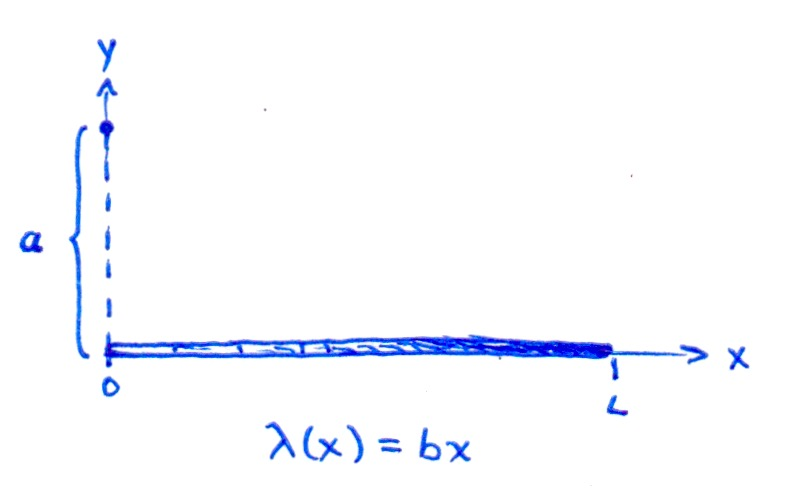A thin bar of length L lies in the xy plane and carries linear charge density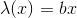, whereranges from 0 to. Calculate the potential at the point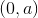on the y-axis.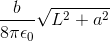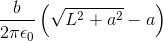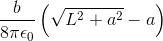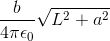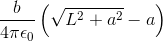Explanation:

Use the linear charge densityand length element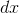, where each point is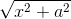from the point. The potential is therefore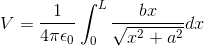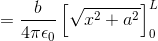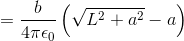### Example Question #11 : Calculating Electric Potential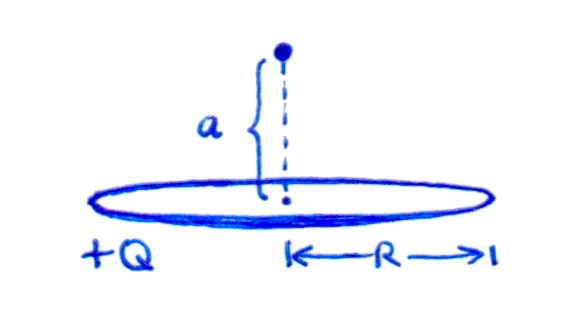A uniformly charged ring of radiuscarries a total charge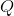. Calculate the potential a distancefrom the center, on the axis of the ring.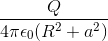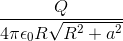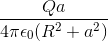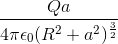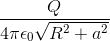Explanation:

Use the linear charge density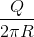and length element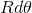. The distance from each point on the ring to the point on the axis is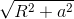. Lastly, integrate over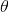fromtoto obtain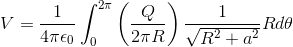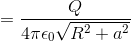### Example Question #181 : Ap Physics CA uniformly charged square frame of side lengthcarries a total charge. Calculate the potential at the center of the square.

You may wish to use the integral: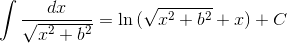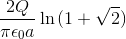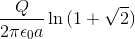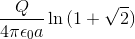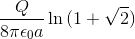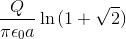Explanation:

Calculate the potential due to one side of the bar, and then multiply this byto get the total potential from all four sides. Orient the bar along the x-axis such that its endpoints are at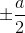, and use the linear charge density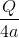. The potential is therefore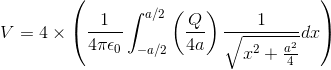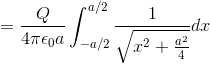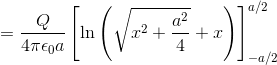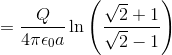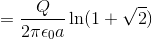### Example Question #1 : Understanding Charge Distributions On Objects

Consider a spherical shell with radiusand charge. What is the magnitude of the electric field at the center of this spherical shell?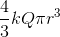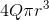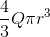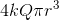Zero

Zero

Explanation:

According to the shell theorem, the total electric field at the center point of a charged spherical shell is always zero. At this point, any electric field lines will result in symmetry, canceling each other out and creating a net field of zero at that point.

### Example Question #1 : Interpreting Electric Charge Diagrams

A charge of unknown value is held in place far from other charges. Its electric field lines and some lines of electric equipotential (V1 and V2) are shown in the diagram.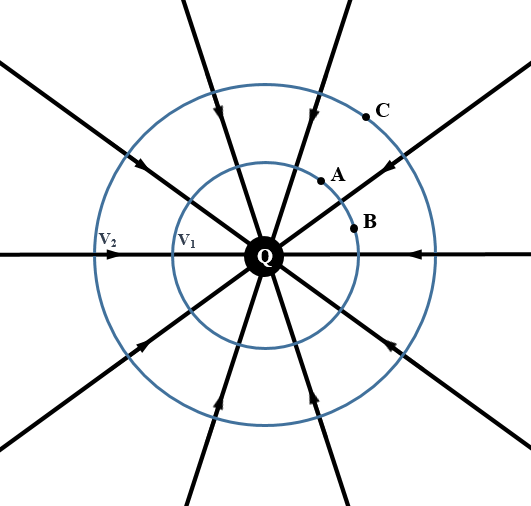A second point charge, known to be negative, is placed at point A in the diagram. In which direction will the second, negative charge freely move?

Toward the original charge Q

It will remain stationary

Toward point A

Toward point C